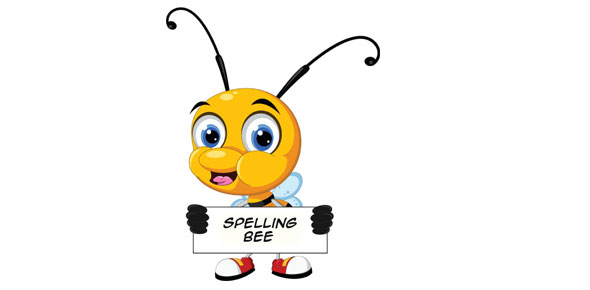# ﻿teach Your Kids The Most Basic Of Mathematics In This Quiz

Approved & Edited by ProProfs Editorial Team
At ProProfs Quizzes, our dedicated in-house team of experts takes pride in their work. With a sharp eye for detail, they meticulously review each quiz. This ensures that every quiz, taken by over 100 million users, meets our standards of accuracy, clarity, and engagement.
| Written by Nicoyuey
N
Nicoyuey
Community Contributor
Total Contribution - 1 | Total attempts - 3,992
Questions: 5 | Attempts: 3,992SettingsMathematics provides an effective way of building mental discipline and encourages logical reasoning and mental rigor. In addition, mathematical knowledge plays a crucial role in understanding the contents of other school subjects such as science, social studies, and even music and art.
Teach your kids the most basic of Mathematics in this quiz

• 1.

### Which of the following means 1 out of 4 equal parts?

• A.

1/2

• B.

1/3

• C.

1/4

• D.

1/5

C. 1/4
Explanation
One out of four equal parts is represented by the fraction 1/4.

Rate this question:

• 2.

### Which of the following numbers is odd?

• A.

0

• B.

2

• C.

13

• D.

24

C. 13
Explanation
The number 13 is odd because it cannot be divided evenly by 2. Odd numbers are any numbers that cannot be divided evenly by 2, leaving a remainder of 1. In this case, 13 divided by 2 equals 6 with a remainder of 1, confirming that it is an odd number.

Rate this question:

• 3.

### How many tens are there in 53?

• A.

0

• B.

3

• C.

5

• D.

50

C. 5
Explanation
There are five tens in the number 53. This is because the digit 5 in the tens place represents 50, which is equivalent to five tens.

Rate this question:

• 4.

### What is 11 minus 3 equal to?

• A.

8

• B.

7

• C.

6

• D.

5

A. 8
Explanation
The correct answer is 8 because when you subtract 3 from 11, you are left with 8.

Rate this question:

• 5.

• A.

• B.

Sum

• C.

Difference

• D.

MinuendBack to top